Next: Parallel processing Up: ALIAS-C++ Previous: Miscellaneous procedures

• La page de J-P. Merlet
• La page Présentation de HEPHAISTOS
• La page "Présentation" de l'INRIA

Subsections

# The ALIAS parser

As it has been seen in the previous chapters the interval evaluation of an equation is highly dependent upon its formulation (for example the evaluation of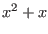may be quite different from the evaluation of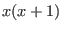for the same range on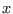). For testing purposes it may therefore be interesting to change the formulation of an equation (for example by using MAPLE), write its analytical form in a file and test what will be the interval evaluation of this equation in this analytical form: this is one objective of the ALIAS parser. Basically this parser takes as input:
• a file, called a formula file, in which is written the analytical form of the equation
• name and ranges for the variables
and will then produces as output the interval evaluation of the equations. In the formula file you may indicate an arbitrary number of equations, each of them being prefixed by eq=. For example

eq=(y^2-1)*z+(2*y*t-2)*x
eq=2+(-10*t+(-10+2*y*t)*y)*y+((4+4*y^2)*z+(4+4*y*t-x*z)*x)*x
eq=(2*y*t-2)*z+(t^2-1)*x
eq=2+(4*x+(4-x*z)*z)*z+((-10+4*z^2)*y+(-10+4*x*z+2*y*t)*t)*t

is a valid formula file in parser format. There is however a limitation on the number of unknowns which cannot exceed 200. Note that the variable names in a formula file may be any name composed with lower and upper cases, integer numbers and underscore, with the constraint that the first character should be a letter. Note also that a MAPLE library enables to produce a formula file directly from MAPLE equations (see section 12.3).

The parser may handle almost any complex analytical equation based on the most classical mathematical functions, using MAPLE notation. Currently you may use the following operators:

• arithmetic operator: "+","-","/","*"," " or "**" (power)
• trigonometric and inverse trigonometric functions: sin, cos, tan, arcsin, arccos, arctan (which may be used with the syntax arctan(x) or arctan(y,x))
• hyperbolic functions: sinh, cosh, tanh, arcsinh, arccosh, arctanh
• mathematical functions: abs (absolute value), log, log10, exp, sqrt
• integer functions: ceil (returns an interval which bounds are the smallest integer greater than or equal to each bound of the box, floor (returns an interval which bounds are the greatest integer less than or equal to each bound of the box), round (returns an interval which bounds are the nearest integer of each bound of the box)
Beside these functions we have added a few more operators:
• Min: this operator takes as input a list of mathematical expression and will return an interval reduced to the minimum value of all the lower bound of the interval evaluation of each term in the list. For example:

Min(3,3*x1,3*x2+x3,4*(x2+x1))

for x1 in [-1,1], x2 in [0,2], x3 in [3,10] will return the interval [-4,-4]
• Max: this operator is similar to Min except it returns the maximal value of the upper bound on the interval evaluation. For the previous example Max will return the interval [16,16]
• MinMax: this operator applied on the list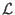will return the interval [Min(), Max()]. In the previous example the interval will be [-4,16]

The parser may also handle intervals. For example you may evaluate an equation in which some coefficients are intervals. In the equation these coefficients should be indicated using the MAPLE notation as in the following example:


INTERVAL(0.1 .. sin(1))


## Using the ALIAS parser in a program

The formula file name will be used as an input in all the procedures described in the following sections. As for the variable you must fill the text variable variable_name_IS defined by


char variable_name_IS;

with the name of you variable and set the integer variable Unknowns_Number_IS to the number of unknowns in your equations as in

strcpy(variable_name_IS,"x");
strcpy(variable_name_IS,"y");
Unknowns_Number_IS=2;

Note that the variable names may be any name composed with lower and upper cases, integer numbers and underscore, with the constraint that the first character should be a letter.

In the following procedures we will then use an interval vector to define the ranges for the unknowns. In this vector the ranges are ordered so that this order will correspond to the order in the declaration of the variable name (in the previous example the first range will be the range for x and the second range will be the range for y).

### Evaluating a single formula

An equation may be evaluated by using the procedure
Evaluate_Interval_With_Parser with the following syntax:

int Evaluate_Interval_With_Parser(
char *texte,INTERVAL_VECTOR &P,int Unknowns,INTERVAL &Value)

The parameters are:
• texte: the name of the file describing the analytical form of the equation
• P: the ranges for the unknowns
• Unknowns: the number of unknowns
• Value: the interval evaluation of the equation
This procedure returns 1 if the equation has been successfully evaluated, 0 otherwise.

### Evaluating multiple equations

Using the parser you may evaluate more than one equation described in a single formula file. Each equation in the file should be prefixed by the symbol eq= like in:

eq=-x1^2-x2+x3
eq=x1^2-x2+x3
eq=round(x3)

All equations in a system of equations may be evaluated by using the procedure
Evaluate_Interval_With_Parser with the following syntax:

int Evaluate_Interval_With_Parser(
char *texte,INTERVAL_VECTOR &P,int Unknowns,int NbEq,INTERVAL_VECTOR &Value)

The parameters are:
• texte: the name of the file describing the analytical form of the equation
• P: the ranges for the unknowns
• Unknowns: the number of unknowns
• NbEq: the number of equations
• Value: the interval evaluation of the equations
This procedure returns 1 if the equations have been successfully evaluated, 0 or a negative number otherwise.

Thus for evaluating the formula described by the formula file toto:


eq=-x1^2-x2+x3
eq=x1^2-x2+x3
eq=round(x3)

for the ranges x1 in [-1,1], x2 in [0,2], x3 in [1.1,2.3] you may use the following piece of code:

INTERVAL_VECTOR P(3);
INTERVAL_VECTOR F(3);
strcpy(variable_name_IS,"x1");
strcpy(variable_name_IS,"x2");
strcpy(variable_name_IS,"x3");
Unknowns_Number_IS=3;

P(1)=INTERVAL(-1,1);//range for x1
P(2)=INTERVAL(0,2);//range for x2
P(3)=INTERVAL(1.1,2.3);//range for x3

Evaluate_Interval_With_Parser("toto",P,3,3,F);
cout<<F<<endl;//interval evaluation of the 3 equations

Instead of evaluating the whole system of equations we may have to evaluate one or more particular equation(s) of the system. The following procedures enable to evaluate efficiently particular equations in a system but before using them we need to do some initialization. This is done by calling once the procedure
Init_Evaluate_Equations_With_Parser with the syntax:

int Init_Evaluate_Equations_With_Parser(char *texte,int *EqStart)

The parameters are:
• texte: the name of the formula file
• EqStart: an array of integer with size equal to the number of equations in the formula file
This procedure returns the number of equations of the system described in the formula file and -1 if the formula file does not exist. It must be used on each formula file with one particular CharEq per file.

After this initialization the following procedure may be used to evaluate one particular equation in a system:


int Evaluate_Equations_With_Parser(char *texte,INTERVAL_VECTOR &P,int Unknowns,

The parameters are:
• texte: the name of the file describing the analytical form of the equation
• P: the ranges for the unknowns
• Unknowns: the number of unknowns
• NbEq: the number of equations
• EqToRead: the number of the equation that has to be evaluated (the equation number start at 1)
• EqStart: the array of integer produced by the initialization procedure for the formula file
• Value: the interval evaluation of the equation EqToRead
This procedure will return 1 on a successful completion. It returns 0 if there was a problem in the evaluation and -1 if the numbering of the equations as indicated in EqToRead is not correct. We may also evaluate more than one equation in a system with:

int Evaluate_Equations_With_Parser(char *texte,INTERVAL_VECTOR &P,int Unknowns,

The parameters are:
• texte: the name of the file describing the analytical form of the equation
• P: the ranges for the unknowns
• Unknowns: the number of unknowns
• NbEq: the number of equations
• EqToRead1: the number of the first equation that has to be evaluated (the equation number start at 1)
• EqToRead2: the number of the last equation that has to be evaluated
• EqStart: the array of integer produced by the initialization procedure for the formula file
• Value: the interval evaluation of the equation EqToRead
This procedure will return 1 on a successful completion. It returns 0 if there was a problem in the evaluation and -1 if the numbering of the equations as indicated in EqToRead1 or EqToRead2 is not correct. We may also use the gradient of the equations to improve the evaluation. The syntax is:

char *gradient,INTERVAL_VECTOR &P, INTERVAL_VECTOR &Value,int Exact)

The parameters are:
• Unknowns: the number of unknowns
• NbEq: the number of equations
• texte: the name of the file describing the analytical form of the equation
• gradient: the name of the parser file giving the gradient matrix of the equations. The order in this file is to define successively the derivative of the first equation with respect to each variable, then the derivative of the second equation and so on
• P: the ranges for the unknowns
• Value: the interval evaluation of the NbEq equations
• Exact: if 0 stop processing the equations as soon as the interval evaluation of the n-th equation does not include 0 (the interval evaluation of the remaining n,..,NbEq equations will be set to 1. If 1 the procedure computes the interval evaluation of all the equations

## Example of use of the parser

The program Evaluation provided in the ALIAS distribution is a simple example of the use of the evaluation through the parser. In its simplest form it takes as first argument a formula file and as second argument a range file (see section 12.2 for the definition of a range file) and print the interval evaluation of all the equations:


Evaluation [formula file] [range file]

A third integer argument n1 may be the number of the equation we want to evaluate (the number start at 1), while if a fourth integer argument n2 is present the evaluation of the equations from n1 to n2 will be printed.

# The generic Solver

A generic solver, called Interval_Solver, based on the parser is available in the ALIAS distribution. The basic arguments of this program is a formula file which defines the equations to be solved and a range file which describe the possible ranges for each of the unknowns in the equations. A line of this range file starts by the name of the variable, followed by two numbers which indicate the range for the variable. For example the following lines define a valid range file

psi 0 0.2
x 1 2.5

If f denotes a formula file and r a range file then you may run:

Interval_Solver -F f -R r

In that case the program will call the procedure Solve_General_Interval12.1 of the ALIAS library.

Instead of using the Solve_General_Interval procedure of ALIAS you may use the
Solve_General_Gradient_Interval procedure which requires the gradient of the equations. The gradient equations are defined in a formula file (e.g. g) and you may then run:


Interval_Solver -F f -G g -R r

Note that the equations in the gradient formula file should be ordered with respect to the unknowns using the same ordering than the one used in the range file. Thus for the range file:

x 1 2.5
y 0 0.2

and the formula file f:

eq=y+3*x
eq=x^2+x*y

denoted eq1, eq2, the first line of the gradient formula file should be deq1/dx, the second line deq1/dy and so on, which will lead to the following gradient file:

eq=3
eq=1
eq=2*x+y
eq=x


Similarly, instead of using the Solve_General_Gradient_Interval procedure of ALIAS you may use the Solve_General_JH_Interval procedure which requires the gradient and hessian of the equations. The hessian equations are defined in a formula file (e.g. h) and you may then run:


Interval_Solver -F f -G g -H h -R r

Note that the hessian formula file should respect the same ordering than for the gradient formula file. For our previous example the hessian file will be:

eq=0
eq=0
eq=0
eq=0
eq=2
eq=1
eq=1
eq=0

Note also that the various formula files may be easily obtained by using the MAPLE procedures described in the next section (12.3).

The result of the computation will be written in a result file. Each line of the result file gives the range for one of the unknowns, using the same ordering than for the range file (in our example the range of x, followed by the range for y). A range is indicated by two reals separated by a blanc character. If instead of range the result file contain the keyword FAILED it means that the solver has been unable to solve the equations. This keyword is followed by an integer which indicate the reason of the failure:

• -1: an insufficient storage space has been defined for the solver
• -4: the number of equation in the formula file is incorrect
The default name for the result file is .result_IS.

Whatever the used solving procedure, some parameters are to be set. The solver will prompt you to give the values of these parameters. If you want to avoid this interactive behavior you may define the parameters in a configuration file and the solver will proceed directly to the solution. A configuration file consists in a list of keywords followed by a value. Most of these keywords refers to parameters in the solving procedure of ALIAS, please refer to the corresponding section for their meaning:

• Result_File name: the result will be stored in the name file instead of the default .result_IS file.
• Iteration integer: the maximum number of boxes that may be used by the algorithm
• Debug integer: the debug level for the solving procedure as indicated by Debug_Level_Solve_General
• AccuracyF real: the epsilonf parameter of the solving procedure
• AccuracyV real: the epsilon parameter of the solving procedure
• Order integer: the Order parameter of the solving procedure
• Stop integer: the Stop parameter of the solving procedure
• MaxSol integer: the maximum number of solutions you are looking for
• Kanto integer: the ApplyKanto parameter of the solving procedure
• DifferenceS real: the Dist parameter of the solving procedure
• Machine_Name char: the name of a computer that will be used during a run of the parallel version of the solver
• Single_Bisection: if you use the solver with the -H or -G option the bisection process will bisect only one variable at a time

## Dealing with inequalities

The generic solver may deal also with inequalities. Here we will add an argument to the generic solver whose syntax will be

-I [inequalities file]

inequalities file is a formula file, called the inequalities file that describe equations in the same manner than a formula file. But the solver will output as solutions only the ranges for which all the equations in the inequalities file may be positive or equal to 0 and eliminate the solutions ranges for which at least one equation is strictly negative. Note that the algorithm stops only according to the values of the equations or the diameter of the ranges and not according to the values of the inequalities.

## Dealing with parametric system

It may also be interesting to investigate the case of parametric systems, i.e. of systems for which some coefficients will be described in analytical form in the formula file, but which are not unknowns. An additional argument for the generic solver will be a file, called the parameter file which will give specific values for the parameter. For example in the equation:

eq1:=x^2-x*cos(y)-x1:

x1 may be considered as a parameter while x, y are the unknowns. Each line of the parameter file indicates a name of a parameter and its value. Thus

x1 1

is a valid parameter file. Then you may use the programs of the MAPLE library (see section 12.3) to generate the parser files for the solver: these programs enable to specify that x1 is not an unknown. The generated files will contain the parameter x1 and if you want to solve a particular occurrence of the parametric system you just have to add an argument to the command line of the generic solver:

-P [parameter file]

and if you want to study another occurrence (for example when the value of x1 is 2) you just have to change the value in the parameter file.

Some specific parameters with special names may also be used to speed up the computation, see section 12.4.4.2.

# MAPLE library for the Interval Solver

All these procedures are available in The lib_IS.m library contains various procedures that may be useful for producing files for the parser. The following MAPLE programs are available:
• cout(eq): estimates the cost of the computation of eq in terms of number of arithmetic operations. This a rather rough approximation: it just compute the total number of addition, multiplication and function calls without any weight between these operators.
• minimal_cout(eq): returns an expression equivalent to eq but with a minimal cost of computation. Basically it consider the expanded form of the equation and compute its computational cost using the cout procedure. Then it consider the Horner forms of the equation using all possible ordering of the unknowns (if you have up to 6 unknowns). The cost of these forms are computed and as soon as a form as a lower cost then it is stored as the potential return for the function. If two forms have equal cost, then the form with the lower number of operations (obtained through the nops procedure) is retained.
• write_equation: this procedure takes as argument an equation or a list of equations and a name. The equation will be written in a minimal form in the formula file defined by the name
• write_gradient_equation: this procedures takes as as argument an equation or a list of equations and a name. The gradient of the equations in minimal form will be written in the formula gradient file defined by the name. If no third argument is provided the ordering of the gradient matrix with respect to the unknowns will be the one provided by the MAPLE procedure indets. A third argument may be provided and should be a list of unknown names which will be used for the ordering of the gradient matrix (i.e. if the list is [x1,x2,x3] for the equation eq the first equation will be deq/dx1, the second deq/dx2..). A fourth argument may be the list of unknowns and is used for parametric system for which the number of undefined variables may be larger than the number of unknowns (otherwise the procedure will return an error message indicating that the equation has more indeterminate than the number of unknowns given in the third argument).
• write_hessian_equation: a similar procedure than for the gradient except it computes the hessian matrix of the equations
An example of the use of this library follows:

eq1:=y**2*z+2*x*y*t-2*x-z:
eq2:=-x**3*z+4*x*y**2*z+4*x**2*y*t+2*y**3*t+4*x**2-10*y**2+4*x*z-10*y*t+2:
eq3:=2*y*z*t+x*t**2-x-2*z:
eq4:=-x*z**3+4*y*z**2*t+4*x*z*t**2+2*y*t**3+4*x*z+4*z**2-10*y*t-10*t**2+2:
write_equation([eq1,eq2,eq3,eq4],"fcap"):
write_hessian_equation([eq1,eq2,eq3,eq4],"hcap",[x,y,z,t]):

The formula file for the 4 equations will be written in the file fcap while the jacobian and hessian will be written in the file gcap, hcap. Note that the range file should define the ranges for the unknowns in the order x,y,z,t.

# The generic analyzer

## Principle

The purpose of the generic analyzer is to try to refine a box and to propose smaller sub-intervals which may contain real roots of the system of equations (which may be algebraic or not). Two types of tools are used for that purpose:
• as soon as one of the equation in the system is algebraic in at least one of the unknowns then the tools described for determining ranges on the roots of an univariate polynomial with interval coefficients are used recursively
• otherwise Kantorovitch theorem is used (if you have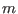equations and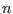unknowns with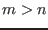, then this theorem is applied only for the firstequations)
More precisely consider the system in two unknowns: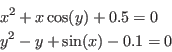In a first step the first equation is considered: it will be considered as a polynomial inwith the interval coefficients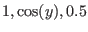. Bounds on the real roots of this polynomial will be computed and the range onwill be updated if necessary. Then the second equation will be considered as a polynomial in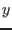with interval coefficients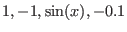Bounds on the real roots of this polynomial will be computed and the range onwill be updated if necessary. After these two steps if a range has been improved we will reiterate the process until no further improvement is obtained.

We will then try to improve the obtained ranges by using Kantorovitch theorem. We consider the middle point of the ranges and test if Kantorovitch is able to determine a ball centered at this point for which Newton method will converge toward the unique solution in the ball. If the answer is positive we will use Newton with as starting point the center of the ball: the result, a point, is stored as one of the result ranges. The intersection of the ball with the initial range will then be computed and if non empty will be stored for further processing.

We may indeed perform an in-depth analysis. The previous process is applied on the initial range and will lead to a set of ranges (possibly to the same ranges than the initial one); we may then bisect the obtained ranges and reiterate the process on the resulting ranges, discarding those for which the interval evaluation for at least one of the equations does not include 0. We define a depth level for the algorithm as the number of bisection steps that will be used in the process (a depth 0 means that no bisection will be done).

## Practical implementation

### The generic analyzer

The purpose of the generic analyzer is to consider as input any system defined in a MAPLE file and an initial set of ranges for the unknowns and to produce as output a set of ranges, strictly included in the initial set, that may contain real roots of the system. The output is presented as a file that can be used directly as input for the generic solver.

A formal-numerical approach has been used to develop this generic analyzer. Basically it takes as input a MAPLE file, called the formula file, which define the analytical form of the equations of the system and a range file in in which are defined both the names of the variables and their initial ranges (a range file may contain more than one set of ranges for the unknowns). The convention in the formula file is to define each equation of the system in the MAPLE file as a sentence prefixed by the key-word eqn where n is the number of the equation starting at 1. For example


eq1:=y**2*z+2*x*y*t-2*x-z:
eq2:=-x**3*z+4*x*y**2*z+4*x**2*y*t+2*y**3*t+4*x**2-10*y**2+4*x*z-10*y*t+2:
eq3:=2*y*z*t+x*t**2-x-2*z:
eq4:=-x*z**3+4*y*z**2*t+4*x*z*t**2+2*y*t**3+4*x*z+4*z**2-10*y*t-10*t**2+2:

is a valid formula file, describing a set of 4 equations. The range file has as many lines as unknowns and each line start by the name of the variable followed by two numbers indicating the range for the variable. For example

x -100 100
y -100 100
z -100 100
t -100 100

is a valid range file for the previous formula file. The analyzer is able to deal with infinity in the ranges: instead of using numbers you may indicate infinity or -infinity. In a first step the analyzer will use MAPLE to produce various intermediary files which are needed for the algorithm to proceed (see section 12.4.4.1). For example it will write in the /tmp/Equation file a description of the system compatible with the syntax used by the parser. Similarly you will find in the /tmp/Gradient_Equation and Hessian_Equation files a description of the gradient and hessian of the system. As soon as all the necessary files have been written, the algorithms described in the previous section will be used.

The result of the analyzer is a file (by default .result_IA) that describe the possibles ranges which may contain real roots of the system. This file may then be used as input for the solver. The analyzer will also produced the .gradient_IA file that indicate the value of the gradient matrices for each of the out ranges.

The analyzer is run by using the syntax:


Interval_Analyzer -F [formula file] [-G] [-H] -R [range file]

In this list of argument only the -F, -R are compulsory. The -G option indicates that you will use the monotonicity of the equations (evaluated through an interval evaluation of the gradient) to improve the interval evaluation of the equations. The -H indicates that you will also use Kantorovitch theorem to isolate the real roots of the equations. In that case if you haveequations andunknowns with, then Kantorovitch will be applied on thefirst equations.

This program will then ask you the maximum number of ranges that may be created by the algorithm, an accuracy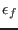on the value of the equations that will be used to stop the computation of the Newton scheme, a minimal value for the maximal diameter for the output ranges (which is not used right now), a depth level, a maximal diameter for the ranges over which Kantorovitch is not used and the value of the debug level (0 if the program is to be run quietly, 1 to see how many boxes are dealt with and 2 for a full debug). Note that if you are using the -H option and haveequations andunknowns, withand if the algorithm returns roots of the system (i.e. the output ranges are reduced to a point) it will mean that these points are guaranteed roots of thefirst equations (you may use the Newton scheme with a loweron these roots and you will still get a root), while the absolute values of the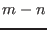remaining equations at these points will be lower thanbut there is no guarantee that these points are effectively roots of these equations.

Alternatively you may use a configuration file to give values for these parameters.

A configuration file consists in a list of keywords followed by a value.

• Result_File name: the result will be stored in the name file instead of the default .result_IA file.
• Iteration integer: the maximum number of boxes that may be produced by the algorithm. Indicating this value is compulsory.
• Debug integer: the debug level (0=no debug, 1=low level debug, 2=full debug)
• AccuracyF real: the epsilonf parameter that will be used to determine a solution if Moore or Kantorovitch succeed.
• Kanto real: we will apply Kantorovitch test as soon as the maximal width of the input ranges is lower than real
• Min_IA real, Mini_IA real: if the change in a variable exceed a threshold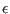we start again the analysis. We give here two values such that Mini_IA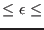Min_IA. The default value is 0.001 for Mini_IA and 0.01 for Min_IA
• Directory name: if you have already processed the equations with MAPLE you may give here the name of the directory where the files resulting from the processing have been written. This avoid to re-process the equations.
• Location_IS name: the location of the library lib_IS.m
• Level integer: the level of bisection you want (from -1, no bisection to any positive number)
• Use_Resultant: the resultant of any two equations which are algebraic in any variable will be computed and will be used to discard input ranges. This option may add a significant amount of time to the pre-preprocessing. If you have a large number of equations you will end up with a large number of equations resulting from the computation of the resultant. You may however use only a subset of these equations by first processing the equations without this option, compute some resultant equations by hand and indicate that they are resultant equation by writing them in parser format in the file /tmp/Resultant, while indicating their number in the file /tmp/DimensionResultant. If you are using the option -G or -H of the analyzer you will have to provide also the gradient equations of the equations you are using in a file called Gradient_Resultant.
• LevelC integer: if the integeris greater or equal to 0 the first test the analyzer will perform is to consider in turn each variable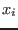and to compute the interval evaluation of the equations withhaving as interval value first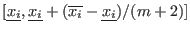and then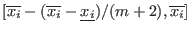. If the interval evaluation of one equation has a constant sign, then the interval forwill be modified to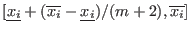in the first case and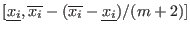in the second case. If one variable at least is modified with an amplitude of modification larger than Mini_IA the process is reiterated. Instead of using this process each time a variable is change we may use it only from time to time. This may be done by setting LevelC to a negative number lower or equal to -10. This number will indicate at the same time the number of variable changes that must occur before using the process and the value ofused for managing the interval. For example a value of -100 will indicate that the process will be used every 10 changes of variable (rint(-LevelC)/10) with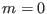(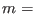10(-LevelC/10-rint(-LevelC)/10)). This process may be computer intensive and therefore the default value of LevelC is -1 so that it is not used.
• UseFullCycle: when using the bound theorems the analyzer will consider in turn each equation and then in turn each variable in which the current equation is algebraic. If an update in a variable has taken place with an amplitude larger than Mini_IA the analyzer will continue until all variables of the current equation has been considered and then will start again the analysis from equation 1. If you put this key-word in your configuration file the analyzer will start again the analysis only when all the equations have been processed. Turning on this option may be interesting if you have used the LevelC option as processing the equations is in general faster than using the combinatory approach: with this option you may have change on a larger number of unknowns which may lead to better result when using the combinatory approach.
For example

Iteration 1000
AccuracyF 0.00001
Debug 0
Kanto 0.5
Level 2
Location_IS /u/ALIAS/Maple

is a valid configuration file. It indicates that at most 1000 ranges may be created (this is not the maximal number of ranges at the end of the algorithm but the maximal number of ranges that may be created at any time in the algorithm), that the accuracy for the Newton scheme is 0.00001, that the algorithm will run quietly, that Kantorovitch will be used as soon as the maximal diameter of the considered range is lower than 0.5 and that the depth level will be 2. We indicate also in which directory is located the library lib_IS. files are located.

You use the configuration file with the syntax:


Interval_Analyzer -F [formula file] -G -H -R [range file] -C
[configuration file]

So a typical analysis and solving process will be done by using the two following command lines (which are basically identical to what is used in the script script_solver provided in the standard distribution of ALIAS):

Interval_Analyzer -F equation.maple -G -H -R range -C conf_analyzer
Interval_Solver -F /tmp/Equation -G /tmp/Gradient_Equation -H
/tmp/Hessian_Equation -R .result_IA -GI .gradient_IA -C conf_solver

You will then have the result of the solution of your system in the file .result_IS.

## Dealing with inequalities

The generic analyzer may deal also with inequalities. Here we will add an argument to the generic analyzer whose syntax will be

Interval_Analyzer -F [formula] [-G -H] -R [range] -I [inequalities]

inequalities is a MAPLE file, called the inequalities file that describe equations in the same manner than a formula file. But the analyzer will output as possible ranges only the ranges for which all the equations in the inequalities file may be positive or equal to 0 and eliminate the ranges for which at least one equation is strictly negative.

## Pre-processing and dealing with parametric equations

### Pre-processing

In some cases it may be interesting to analyze a given system of equations with various initial input ranges. In the current state the system will be processed again and again for each input ranges. If this system is large this result in unnecessary computation time. When processing for the first time a system the generic analyzer will produce intermediary files in parser format in the /tmp directory. If you use the -G and -H option of the analyzer the intermediary files will be:
• Equation: the system of equation
• Hessian_Equation: the hessian equations of the system
• DimensionEq: the dimension file i.e. a file which contains a list of integer which indicates the degree of the first equation in the first unknown, in the second unknowns,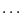, then a similar list for the second equation, and so on
• CoeffEq-: the coefficients of the equationof the system in the unknown number• Gradient_CoeffEq-: the gradient of the coefficients of the equationof the system in the unknown numberNote also that you may have to be careful when stopping the analyzer when it is generating these files. Indeed on some systems these files may be read-write protected even for the owner. If you run again the analyzer MAPLE will not be able to write the same files and will produce an error message: you will then need to remove by hand each of the created files and start again.

As soon as these files have been generated once it is not necessary to re-compute them. To indicate that these quantities have been already processed it is is sufficient to include the following sentence in the configuration file:


Directory name

Instead of computing these files the analyzer will look for them in the directory name. Thus for example after running the analyzer for the first time without this sentence in the configuration file you may analyze the same system without processing the file by indicating in your configuration file:

Directory /tmp


### Parametric equations

It may also be interesting to use the pre-processing for parametric systems, i.e. for systems for which some coefficients will be described in analytical form in the MAPLE formula file, but which are not unknowns. An additional argument for the generic analyzer will be a file, called the parameter file which will give specific values for the parameter. For example in the equation:

eq1:=x^2-x*cos(y)-x1:

x1 may be considered as a parameter. On the first run of the analyzer the intermediary files described in the previous section will be generated and at run time the value of x1 will be substituted by the value indicated in the parameter file. After this first run and if you want to analyze the system with another value for x1 you may use the pre-processing mechanism described in the previous section and just change the value of x1 in the parameter file. See section 12.4.6.1 for an example of the analysis of parametric system.

Each line of the parameter file indicates a name of a parameter and its value. Thus


x1 1

is a valid parameter file. To indicate a parameter file to the analyzer use the -P argument followed by the name of the parameter file.

A special case of parameter may be used to speed up the computation. Assume that you have a system with large numerical constants (by "large" we mean that the string representing the numerical values has a large number of characters). Each evaluation of your system will require first to read the strings representing the numerical constants and then to convert it in a float number. It may therefore be interesting to store once the constants values and to substitute them in the analytical form of the equations by symbols which may be attached to these values. This may be done by substituting the numerical constants by symbols of the form _IAwhereis an integer starting from 0. These parameters have then to be stored in a parameter file. Thus, for example, consider the equations:


eq1:=1.12345678901*x1+4.563213456789222:

Using MAPLE you may easily change this equation to:

eq1:=_IA0*x1+_IA1:

and store the values in a parameter file:

_IA0 1.12345678901
_IA1 4.563213456789222

When reading the parameter file the program will store in a special array the value of the parameters whose name start by the symbol _IA. Then, when the parser find a symbol starting with this symbol it will determine the number following this keyword (which is the value of the index in the parameter table) and will just substitute the corresponding value found in the parameter table. Hence when evaluating an expression the parser will have to read less character and avoid unnecessary conversion from a string to a double. Note that these parameters must be numbered in sequence, starting from 0.

## Errors and Debug

The analyzer may generate the following error code:
• -1: too many ranges have been generated during the algorithm. Note that this test is only approximate.
• -2: there is an error in the files generated for the parser
• -4: the number of equations in your system is not correct (this should not occur with the generic analyzer but may happen if you use it in a program)
• -11: the parameters equation_processed of the analyzer is not 0 or 1 (cannot occur for the generic analyzer)
• -20: the dimension file cannot be opened
• -40: the degree of the equations in the various unknowns is 0 (should not occur for the generic analyzer)
You may get some debug information by setting the variable
Debug_Level_Solve_General_Interval to 1 (minimal level of debug information) or 2 (full debug).

## Examples

### Non algebraic equations

Let consider the following system

eq1:=x^2-x*cos(y)-1:
eq2:=y^2-cos(x)-1:

for which we want to determine if real roots exist in the range [-5,5], [-5,5]. With a depth level 0 (no bisection is done) the analyzer proposes the following ranges as possibly containing a real root:

Range 1( x y)
[-0.86812856880248989722,-0.86812856880248989722]
[-1.28306500467802298,-1.28306500467802298]
Range 2( x y)
[1.219833908062562422,1.219833908062562422]
[-1.1592247392497665448,-1.1592247392497665448]
Range 3( x y)
[1.2198350997243703198,1.2198367316341245381]
[-1.159223547587958647,-1.1592195879408853099]
Range 4( x y)
[-0.86812856880248978619,-0.86812856880248978619]
[1.28306500467802298,1.28306500467802298]
Range 5( x y)
[1.219833908062562422,1.219833908062562422]
[1.1592247392497667668,1.1592247392497667668]
Range 6( x y)
[1.2198352388536839452,1.2198367316341249822]
[1.1592195879408850878,1.1592236867169092296]

this 6 ranges, 4 are reduced to point and are therefore a result of the application of the Newton scheme. The other 4 ranges are very close to one of the solution, but the algorithm has not been able to eliminate them. With a depth level of 1 the algorithm proposes directly the four solutions of this system:

Range 1( x y)
[-0.86812741994331155126,-0.86812741994331155126]
[-1.2830653469203250339,-1.2830653469203250339]
Range 2( x y)
[1.2198339926936523359,1.2198339926936523359]
[-1.1592245852349318813,-1.1592245852349318813]
Range 3( x y)
[-0.86812741994331166229,-0.86812741994331166229]
[1.2830653469203250339,1.2830653469203250339]
Range 4( x y)
[1.219833901899016082,1.219833901899016082]
[1.159224777165462017,1.159224777165462017]

Note that the initial range [-5,5] may be largely expanded without modifying the result and with only a low amount of additional computation time. For example for the range [-1000,1000] for both variables the computation time remains the same.

The previous system may be considered as a special occurrence of the system:


eq1:=x^2-x*cos(y)-x1:
eq2:=y^2-cos(x)-1:

where the parameter x1 has value 1. This special case of the generic system may be analyzed by indicating in a parameter file:

x1 1

Now just by changing the value of x1 in this file to

x1 2

and modifying the configuration file to include the sentence:

Directory /tmp

we will get the solutions:

Range 1( x y)
[-1.2271026453420417202,-1.2271026453420417202]
[-1.1562745250557344701,-1.1562745250557344701]
Range 2( x y)
[1.757647484313144215,1.757647484313144215]
[-0.90234927430355182931,-0.90234927430355182931]
Range 3( x y)
[-1.2271026453420417202,-1.2271026453420417202]
[1.1562722309470763182,1.1562722309470763182]
Range 4( x y)
[1.757647484313144215,1.757647484313144215]
[0.90234927430355205136,0.90234927430355205136]


### Using the generic analyzer in a program

You may use directly the analyzer in a program using the following syntax:
int Equation_Analyzer(int Dimension,int Dimension_Eq,int MaxBox,
char *inequalities_file,char *dimension_file,char *coeff_file,
int method,int nb_inequalities,int equation_processed,
INTERVAL_VECTOR &Input,
double Acc_Var,double Acc_Eq,double Acc_Kanto,
int Depth_Level,
INTERVAL_MATRIX &Range,
int *Nb_Range);

with:

• Dimension: the number of unknowns in the system
• Dimension_Eq: the number of equations in the system
• MaxBox: the maximum number of ranges that may be produced at any step of the algorithm
• formula_file: if the system has already been processed the name of the file which contain the equations in parser format
• gradient_file: if the system has already been processed the name of the file which contain the gradient equations in parser format
• hessian_file: if the system has already been processed the name of the file which contain the gradient equations in parser format
• inequalities_file: if the system has already been processed the name of the file which contain the inequalities equations in parser format. The number of inequalities must be given in nb_inequalities
• dimension_file: the name of the dimension file, i.e. the file which contain the degree of the equations in the unknowns (see section 12.4.4.1). If this string has 0 length the analyzer will assume that you have filed the integer array Dimen_IA(Dimension_Eq,Dimension with the appropriate number: for example Dimen_IA(1,2) should contain the degree of the first equation in the second variable (the order of the variable is their order of occurrence in the string array that contain their names).
• coeff_file: the base-name for the files containing the coefficients of the equations. For example
coeff_file1-2 must contain the coefficients of the first equation considered as a univariate polynomial in the second variable
• gradient_coeff_file: the base-name for the files containing the gradient of the coefficients of the equations. For example
gradient_coeff_file1-2 must contain the gradient of the coefficients of the first equation considered as a univariate polynomial in the second variable
• method: an integer describing the method you are using for evaluating the equation:
• 0: straightforward interval evaluation
• 1: evaluation using the gradient of the equation
• 2: use Kantorovitch and Newton scheme to determine roots of the system and intervals containing unique roots of the system.
• nb_inequalities: the number of inequalities constraints you have
• equation_processed: an integer which may be 0 or 1.
• 0: you have not processed your system. The analyzer will consider that the argument formula_file is a MAPLE program that contain the description of your equations. It will then create all the necessary intermediate files (see section 12.4.4.1). Note that in that case all the string files of this procedure will be substituted by the names of the corresponding intermediary files. The storage space of these strings must therefore be sufficient.
• 1: the system has already been processed and the name indicated in the arguments of this procedure are parser files
• Input: the initial set of ranges for the unknowns
• Acc_Var: unused at this time
• Acc_Eq: if method is 2 the threshold under which an equation will be considered to be equal to 0 in the Newton scheme
• Acc_Kanto: if method is 2, the maximal diameter in a set of ranges under which we start applying Kantorovitch. A typical value is 0.5
• Depth_Level: the level of bisection that will be used by the analyzer (from 0 to some positive value)
• Range: the sets of ranges produced by the algorithm
• Nb_Range: the number of sets in Range

Beside these arguments you must fill the string array variable_name_IS with the name of the unknowns. If you have a parametric system you must indicate the name of the parameters in the string array parameter_name_IS, their values in the array of doubles parameter_value_IS and set the integer variable Nb_Parameter_IA to the number of parameters.

# Using the parser programs

The parser programs are available in the library lib_Parser.a. You will have to include the following header files:


If you are planing to use the analyzer you must include the library lib_UP.a. In your program you must avoid to use variables whose name include the symbols _IA and _IS.

The following programs are equivalent to the standard ALIAS programs except that they use the parser.


int Kantorovitch_Analyzer(int Dimension,
char *Hessian_File,
VECTOR &Input,double *eps);

int Newton(int Dimension,
char * TheIntervalFunction_File,
VECTOR &Input,double Accuracy,int Max_Iter,VECTOR &Residu);

int Solve_General_Interval(int Dimension_Var,int Dimension_Eq,
char * TheIntervalFunction_File,
INTERVAL_VECTOR & TheDomain,
int Order,
int Iteration,
int Stop_First_Sol,
double Accuracy_Variable,
double Accuracy,
double Dist_Sol_Diff,
INTERVAL_MATRIX & Solution,int Nb_Max_Solution,
int nb_inequalities,char *Ineq_File);
char * TheIntervalFunction_File,
INTERVAL_VECTOR & TheDomain,
int Order,
int Iteration,
int Stop_First_Sol,
double Accuracy_Variable,
double Accuracy,
double Dist_Sol_Diff,
INTERVAL_MATRIX & Solution,int Nb_Max_Solution,
int nb_inequalities,char *Ineq_File);
char * TheIntervalFunction_File,
INTERVAL_VECTOR & TheDomain,
int Order,
int Iteration,
int Stop_First_Sol,
double Accuracy_Variable,
double Accuracy,
double Dist_Sol_Diff,
INTERVAL_MATRIX & Solution,int Nb_Max_Solution,
int nb_inequalities,
char *Ineq_File);
int Solve_General_JH_Interval(int Dimension,int Dimension_Eq,
char * TheIntervalFunction_File,
char * Hessian_File,
INTERVAL_VECTOR & TheDomain,
int Order,
int Iteration,
int Stop_First_Sol,
double Accuracy_Variable,
double Accuracy,
INTERVAL_MATRIX & Solution,
INTEGER_VECTOR & Is_Kanto,
int Apply_Kanto,
int Nb_Max_Solution,
int nb,char *file);Next: Parallel processing Up: ALIAS-C++ Previous: Miscellaneous procedures